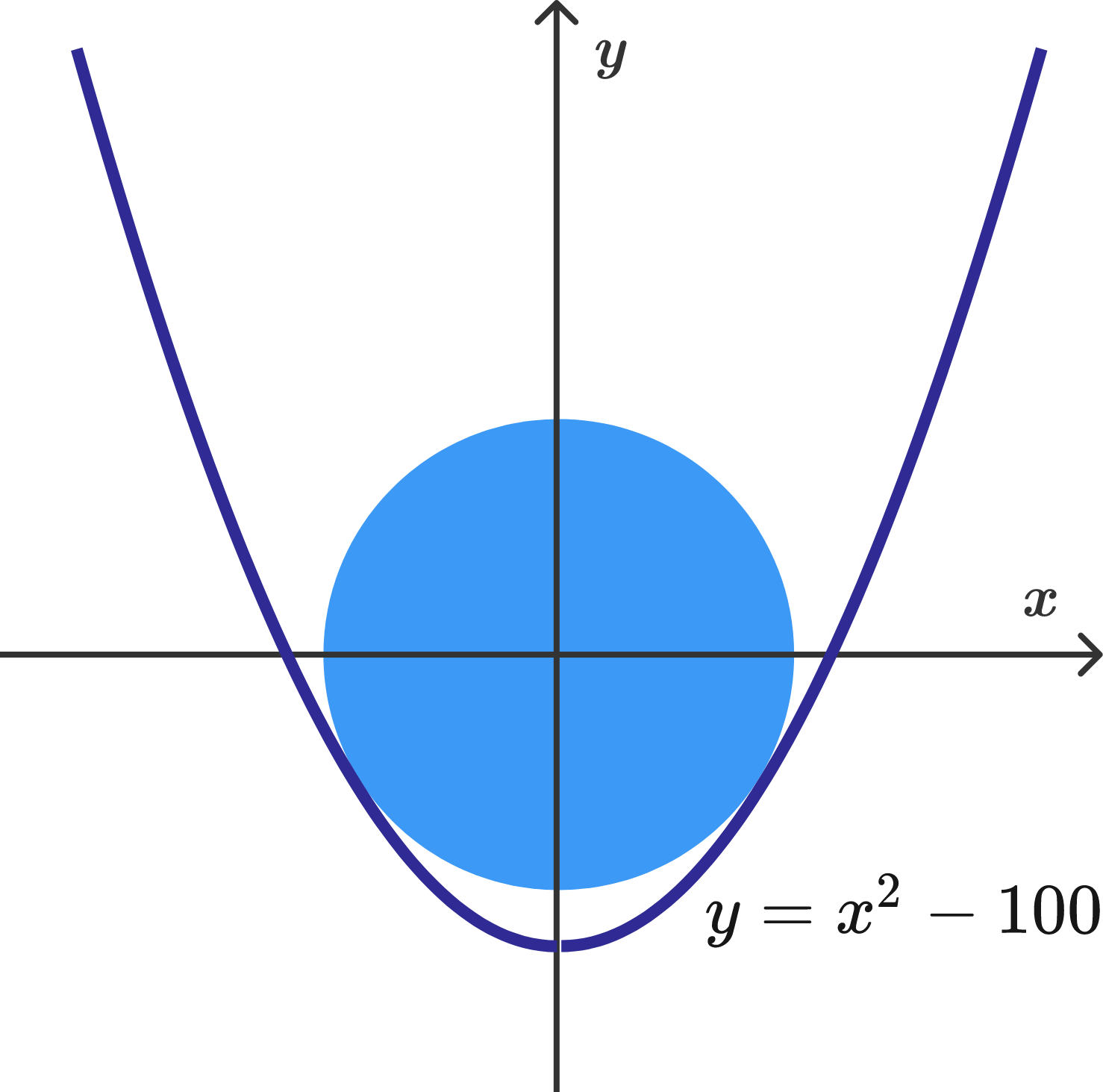# Circle Inscribed in a Parabola

Geometry Level 4

The area of a circle centered at the origin, which is inscribed in the parabola $y=x^2-100,$ can be expressed as $\frac{a}{b} \pi,$ where $a$ and $b$ are coprime positive integers. What is the value of $a+b$?×